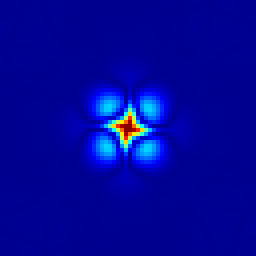/** # Geostrophic adjustment This test case was originally proposed by [Leroux et al., 1998](#leroux1998) and [Dupont, 2001](#dupont2001) and is also discussed in [Popinet & Rickard, 2007](#popinet2007), section 6.1. We consider the geostrophic adjustment problem studied by [Dupont](#dupont2001) and [Le Roux et al.](#leroux1998). A Gaussian bump $$\eta ( x, y ) = \eta_0 e^{^{- \frac{x^2 + y^2}{R^2}}}$$ is initialised in a 1000 $\times$ 1000 km, 1000 m deep square basin. A reduced gravity $g = 0.01$ m/s$^2$ is used to approximate a 10 m-thick stratified surface layer. On an $f$-plane the corresponding geostrophic velocities are given by \begin{aligned} u ( x, y ) & = \frac{2 g \eta_0 y}{f_0 R^2} e^{- \frac{x^2 + y^2}{R^2}},\\ v ( x, y ) & = - \frac{2 g \eta_0 x}{f_0 R^2} e^{- \frac{x^2 + y^2}{R^2}}, \end{aligned} where $f_0$ is the Coriolis parameter. Following Dupont we set $f_0 = 1.0285 \times 10^{- 4}$ s$^{- 1}$, $R = 100$ km, $\eta_0 = 599.5$ m which gives a maximum geostrophic velocity of 0.5 m/s. In the context of the linearised shallow-water equations, the geostrophic balance is an exact solution which should be preserved by the numerical method. In practice, this would require an exact numerical balance between terms computed very differently: the pressure gradient and the Coriolis terms in the momentum equation. If this numerical balance is not exact, the numerical solution will adjust toward numerical equilibrium through the emission of gravity-wave noise which should not affect the stability of the solution. This problem is thus a good test of both the overall accuracy of the numerical scheme and its stability properties when dealing with inertia--gravity waves. We note in particular that a standard A-grid discretisation would develop a strong computational-mode instability in this case. Also, as studied by Leroux et al, an inappropriate choice of finite-element basis functions will result in growing gravity-wave noise. ~~~gnuplot Evolution of the maximum error on the surface height for the geostrophic adjustment problem. set xlabel 'Time (days)' set ylabel 'Maximum error on surface height (m)' plot 'log' index 'Beta = 0' u 1:2 w l t '' ~~~Evolution of the surface-height error field. From left to right: t = 1.157, 2.315, 3.472, 4.630, 17.361 days.
A variant of this test case uses a $\beta$-plane approximation for the Coriolis parameter: $f = f_0 + \beta y$ with $\beta = 1.607\times 10^{-11}$ m^-1^s^-1^. The numerical energy dissipation is displayed below. Note that this looks larger than for the Gerris equivalent case (as published in [Popinet & Rickard, 2007](#popinet2007)) because the proper reference for (available) potential energy is used here, which was not the case for the Gerris case. ~~~gnuplot Evolution of the total energy for the $\beta$-plane geostrophic adjustment problem. set ylabel 'Normalised energy' set key center right plot 'log' index 'Beta = 1.607e-11' u 1:($3 +$4) w l t 'Total', \ 'log' index 'Beta = 1.607e-11' u 1:4 w l t 'Potential', \ 'log' index 'Beta = 1.607e-11' u 1:3 w l t 'Kinetic' ~~~ ## References ~~~bib @Article{popinet2007, author = {S. Popinet and G. Rickard}, title = {A tree-based solver for adaptive ocean modelling}, journal = {Ocean Modelling}, year = {2007}, number = {16}, pages = {224-249}, url = {http://gfs.sf.net/ocean.pdf} } @phdthesis{dupont2001, author = {F. Dupont}, title = {Comparison of numerical methods for modelling ocean circulation in basins with irregular coasts}, year = {2001}, school = {McGill University, Montreal}, url = {http://basilisk.fr/dupont2001.pdf} } @article{leroux1998, title={Finite elements for shallow-water equation ocean models}, author={Le Roux, Daniel Y and Staniforth, Andrew and Lin, Charles A}, journal={Monthly Weather Review}, volume={126}, number={7}, pages={1931--1951}, year={1998} } ~~~ ## See also * [Same test with Gerris](http://gerris.dalembert.upmc.fr/gerris/tests/tests/geo.html) */ #include "grid/multigrid.h" #include "layered/hydro.h" #include "layered/implicit.h" double F0 = 0., Beta = 0.; #define F0() (F0 + Beta*y) #include "layered/coriolis.h" #define H0 1000. #define R0 100e3 #define ETA0 599.5 int main() { size (1000e3); origin (-L0/2., -L0/2.); init_grid (64); G = 0.01; F0 = 1.0285e-4; // TOLERANCE = 1e-6; linearised = true; DT = 1000; // theta_H = 0.55; fprintf (stderr, "# Beta = %g\n", Beta); run(); Beta = 1.607e-11; fprintf (stderr, "\n\n# Beta = %g\n", Beta); run(); } scalar h1[]; event init (i = 0) { foreach() { zb[] = - H0; // this is important to define the reference level // for (available) potential energy h[] = h1[] = H0 + ETA0*exp (-(x*x + y*y)/(sq(R0))); u.x[] = 2.*G*ETA0*y/(F0*sq(R0))*exp (-(x*x + y*y)/(sq(R0))); u.y[] = - 2.*G*ETA0*x/(F0*sq(R0))*exp (-(x*x + y*y)/(sq(R0))); } } scalar e[]; double error() { double max = 0.; foreach(reduction(max:max)) { e[] = fabs (h1[] - h[]); if (e[] > max) max = e[]; } return max; } typedef struct { double ke, pe; } Energy; Energy energy() { double KE = 0., PE = 0.; foreach(reduction(+:KE) reduction(+:PE)) { KE += h[]*(sq(u.x[]) + sq(u.y[]))/2.*dv(); PE += G*sq(eta[])/2.*dv(); } return (Energy){KE, PE}; } event movie (i += 10) { output_ppm (e, file = "e.mp4", n = 256, spread = -1); } event logfile (i += 1; t <= 20.*86400) { static Energy E0 = {0.,0.}; Energy E = energy(); if (i == 0) E0 = E; double Etot0 = E0.ke + E0.pe; fprintf (stderr, "%g %g %.8g %.8g %g\n", t/86400., error(), E.ke/Etot0, E.pe/Etot0, dt); } event plots (i = 100; i += 100) { if (Beta == 0.) { char s; sprintf (s, "e-%g.png", t/86400.); output_ppm (e, file = s, spread = -1, n = 256); } }